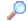Search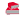Directory

Desktop Functions:

Smart Device Functions: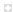Glossary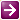Show Recent ChangesSubscribe (RSS)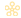Misc. Pages

Summary
Represents the image section header format.

#### C# Definition:

[StructLayout(LayoutKind.Explicit)]
{
[FieldOffset(0)]  public fixed byte Name;
[FieldOffset(8)]  public UInt32 VirtualSize;
[FieldOffset(16)] public UInt32 SizeOfRawData;
[FieldOffset(20)] public UInt32 PointerToRawData;
[FieldOffset(24)] public UInt32 PointerToRelocations;
[FieldOffset(28)] public UInt32 PointerToLinenumbers;
[FieldOffset(32)] public UInt16 NumberOfRelocations;
[FieldOffset(34)] public UInt16 NumberOfLinenumbers;
[FieldOffset(36)] public DataSectionFlags Characteristics;

public string Section
{
get { return new string(Name); }
}
}
[Flags]
public enum DataSectionFlags : uint
{
/// <summary>
/// Reserved for future use.
/// </summary>
TypeReg = 0x00000000,
/// <summary>
/// Reserved for future use.
/// </summary>
TypeDsect = 0x00000001,
/// <summary>
/// Reserved for future use.
/// </summary>
/// <summary>
/// Reserved for future use.
/// </summary>
TypeGroup = 0x00000004,
/// <summary>
/// The section should not be padded to the next boundary. This flag is obsolete and is replaced by IMAGE_SCN_ALIGN_1BYTES. This is valid only for object files.
/// </summary>
/// <summary>
/// Reserved for future use.
/// </summary>
TypeCopy = 0x00000010,
/// <summary>
/// The section contains executable code.
/// </summary>
ContentCode = 0x00000020,
/// <summary>
/// The section contains initialized data.
/// </summary>
ContentInitializedData = 0x00000040,
/// <summary>
/// The section contains uninitialized data.
/// </summary>
ContentUninitializedData = 0x00000080,
/// <summary>
/// Reserved for future use.
/// </summary>
/// <summary>
/// The section contains comments or other information. The .drectve section has this type. This is valid for object files only.
/// </summary>
/// <summary>
/// Reserved for future use.
/// </summary>
TypeOver = 0x00000400,
/// <summary>
/// The section will not become part of the image. This is valid only for object files.
/// </summary>
/// <summary>
/// The section contains COMDAT data. For more information, see section 5.5.6, COMDAT Sections (Object Only). This is valid only for object files.
/// </summary>
/// <summary>
/// Reset speculative exceptions handling bits in the TLB entries for this section.
/// </summary>
NoDeferSpecExceptions = 0x00004000,
/// <summary>
/// The section contains data referenced through the global pointer (GP).
/// </summary>
RelativeGP = 0x00008000,
/// <summary>
/// Reserved for future use.
/// </summary>
MemPurgeable = 0x00020000,
/// <summary>
/// Reserved for future use.
/// </summary>
Memory16Bit = 0x00020000,
/// <summary>
/// Reserved for future use.
/// </summary>
MemoryLocked = 0x00040000,
/// <summary>
/// Reserved for future use.
/// </summary>
/// <summary>
/// Align data on a 1-byte boundary. Valid only for object files.
/// </summary>
Align1Bytes = 0x00100000,
/// <summary>
/// Align data on a 2-byte boundary. Valid only for object files.
/// </summary>
Align2Bytes = 0x00200000,
/// <summary>
/// Align data on a 4-byte boundary. Valid only for object files.
/// </summary>
Align4Bytes = 0x00300000,
/// <summary>
/// Align data on an 8-byte boundary. Valid only for object files.
/// </summary>
Align8Bytes = 0x00400000,
/// <summary>
/// Align data on a 16-byte boundary. Valid only for object files.
/// </summary>
Align16Bytes = 0x00500000,
/// <summary>
/// Align data on a 32-byte boundary. Valid only for object files.
/// </summary>
Align32Bytes = 0x00600000,
/// <summary>
/// Align data on a 64-byte boundary. Valid only for object files.
/// </summary>
Align64Bytes = 0x00700000,
/// <summary>
/// Align data on a 128-byte boundary. Valid only for object files.
/// </summary>
Align128Bytes = 0x00800000,
/// <summary>
/// Align data on a 256-byte boundary. Valid only for object files.
/// </summary>
Align256Bytes = 0x00900000,
/// <summary>
/// Align data on a 512-byte boundary. Valid only for object files.
/// </summary>
Align512Bytes = 0x00A00000,
/// <summary>
/// Align data on a 1024-byte boundary. Valid only for object files.
/// </summary>
Align1024Bytes = 0x00B00000,
/// <summary>
/// Align data on a 2048-byte boundary. Valid only for object files.
/// </summary>
Align2048Bytes = 0x00C00000,
/// <summary>
/// Align data on a 4096-byte boundary. Valid only for object files.
/// </summary>
Align4096Bytes = 0x00D00000,
/// <summary>
/// Align data on an 8192-byte boundary. Valid only for object files.
/// </summary>
Align8192Bytes = 0x00E00000,
/// <summary>
/// The section contains extended relocations.
/// </summary>
/// <summary>
/// The section can be discarded as needed.
/// </summary>
/// <summary>
/// The section cannot be cached.
/// </summary>
MemoryNotCached = 0x04000000,
/// <summary>
/// The section is not pageable.
/// </summary>
MemoryNotPaged = 0x08000000,
/// <summary>
/// The section can be shared in memory.
/// </summary>
MemoryShared = 0x10000000,
/// <summary>
/// The section can be executed as code.
/// </summary>
MemoryExecute = 0x20000000,
/// <summary>
/// The section can be read.
/// </summary>
/// <summary>
/// The section can be written to.
/// </summary>
MemoryWrite = 0x80000000
}

#### VB Definition:

' use struct with LayoutKind.Explicit Attribute to simulate the union in C/C++
<StructLayout(LayoutKind.Explicit)> _
<FieldOffset(0)> _
<FieldOffset(0)> _
Public VirtualSize As System.UInt32
End Structure

<StructLayout(LayoutKind.Sequential)> _
<MarshalAs(UnmanagedType.ByValTStr, SizeConst:=8)> _
Public Name As String
Public SizeOfRawData As UInt32
Public PointerToRawData As UInt32
Public PointerToRelocations As UInt32
Public PointerToLinenumbers As UInt32
Public NumberOfRelocations As UInt16
Public NumberOfLinenumbers As UInt16
Public Characteristics As UInt32
End Structure

None.

#### Notes:

None.

Documentation

Do you have...

• corrections to the existing content?
• alternate definitions?
• additional languages you want to include?

Select "Edit This Page" on the right hand toolbar and edit it! Or add new pages containing any supporting types needed.Access PInvoke.net directly from VS:Terms of UseEdit This PageFind ReferencesShow Printable Version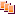Revisions Show changes